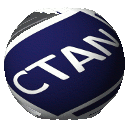# pst-func: plotting special mathematical functions

• psBezier
• Chebyshev polynomials
• psPolynomial (with derivations)
• psBernstein (with envelope option)
• psFourier
• psBessel
• psSi and pssi (integral sin)
• psCi and psci (integral cosin)
• psIntegral
• psCumIntegral
• psConv
• psGauss
• psPoisson
• psBinomial
• psBinomialN
• psFDist for F-distribution
• psChiIIDist
• psTDist for Student's t-distribution
• psNonCentralTDist for noncentral Student's t-distribution
• psWeibull for Weibull distribution
• psLame (Lamé Curve - a superellipse)
• psLorenz
• psThomae (popcorn function)
• psWeierstrass (original and modified)
• psplotImp (plotting implicit defined functions)
• psVolume (rotating f(x) around the x-axis)
• psPrintValue

%% This program can be redistributed and/or modified under the terms %% of the Project Public License Distributed from CTAN archives %% in directory macros/latex/base/lppl.txt.

Save the files pst-func.sty|pro|tex in a directory, which is part of your local tree. The pro file should go into \$TEXMF/dvips/pstricks/ Then do not forget to run texhash to update this tree.

pst-func needs pst-plot (pstricks-add) and pstricks, which should be part of your local installation, otherwise get it from a CTAN server, http://mirror.ctan.org

PSTricks is PostScript Tricks, the documentation cannot be run with pdftex, use lualatex or the sequence latex->dvips->ps2pdf or pdflatex with package auto-pst-pdf or xelatex or lualatex (preferred).

hvoss@tug.org

%% \$Id: README 897 2014-03-21 08:06:41Z herbert \$

## pst-func – PSTricks package for plotting mathematical functions

The package is built for use with PSTricks. It provides macros for plotting and manipulating various mathematical functions:

• polynomials and their derivatives f(x)=an*x^n+an-1*x^(n-1)+...+a0 defined by the coefficients a0 a1 a2 ... and the derivative order;
• the Fourier sum f(x) = a0/2+a1cos(omega x)+...+b1sin(omega x)+... defined by the coefficients a0 a1 a2 ... b1 b2 b3 ...;
• the Bessel function defined by its order;
• the Gauss function defined by sigma and mu;
• Bézier curves from order 1 (two control points) to order 9 (10 control points);
• the superellipse function (the Lamé curve);
• Chebyshev polynomials of the first and second kind;
• the Thomae (or popcorn) function;
• the Weierstrass function;
• various integration-derived functions;
• normal, binomial, poisson, gamma, chi-squared, student’s t, F, beta, Cauchy and Weibull distribution functions and the Lorenz curve;
• the zeroes of a function, or the intermediate point of two functions;
• the Vasicek function for describing the evolution of interest rates; and
• implicit functions.

The plots may be generated as volumes of rotation about the X-axis, as well.

 Package pst-func Home page https://tug.org/PSTricks/main.cgi/ Support https://tug.org/mailman/listinfo/pstricks Repository https://archiv.dante.de/~herbert/TeXnik/ Version 1.02 Licenses The LaTeX Project Public License Maintainer Herbert Voß Contained in TeX Live as pst-funcMiKTeX as pst-func Topics Graphics plot functionGraphics useMathsPSTricks